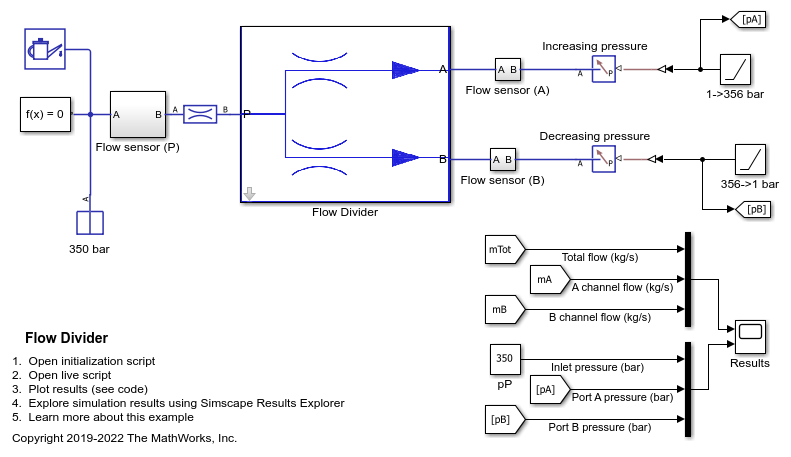# Flow Divider

This example shows a simple way of modeling of the flow divider and using it with the load harness. The flow divider helps in dividing the input flow in desired percentage when the loads connected to the two lines are unequal. Flow divider allows flow only in forward direction. Therefore when it is used with the loads where unloading is done through reverse flows, then it should be used in conjunction with the direction control valves.

### Model

The following figure shows the model of a flow divider with the test harness. The output of the model is the plot of flow through the channels A and B for a given input flow.### Simulation Results from Simscape Logging

This script generates a plot of flow through the channels A and B for a given input flow.